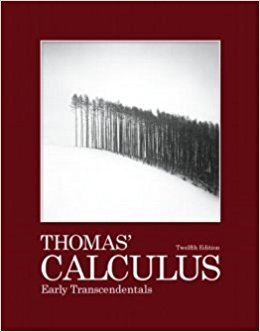×
Log in to StudySoup
Get Full Access to Calculus - Textbook Survival Guide
Join StudySoup for FREE
Get Full Access to Calculus - Textbook Survival Guide

Already have an account? Login here
×
Reset your password

# Solutions for Chapter 2: Limits and Continuity## Full solutions for Thomas' Calculus Early Transcendentals | 12th Edition

ISBN: 9780321588760Solutions for Chapter 2: Limits and Continuity

Solutions for Chapter 2
4 5 0 384 Reviews
28
2
##### ISBN: 9780321588760

Since 56 problems in chapter 2: Limits and Continuity have been answered, more than 10713 students have viewed full step-by-step solutions from this chapter. Thomas' Calculus Early Transcendentals was written by and is associated to the ISBN: 9780321588760. Chapter 2: Limits and Continuity includes 56 full step-by-step solutions. This textbook survival guide was created for the textbook: Thomas' Calculus Early Transcendentals, edition: 12. This expansive textbook survival guide covers the following chapters and their solutions.

Key Calculus Terms and definitions covered in this textbook
• Bounded below

A function is bounded below if there is a number b such that b ? ƒ(x) for all x in the domain of f.

• Convergence of a sequence

A sequence {an} converges to a if limn: q an = a

• End behavior asymptote of a rational function

A polynomial that the function approaches as.

• Expanded form

The right side of u(v + w) = uv + uw.

• Factor

In algebra, a quantity being multiplied in a product. In statistics, a potential explanatory variable under study in an experiment, .

• Frequency (in statistics)

The number of individuals or observations with a certain characteristic.

• Grapher or graphing utility

Graphing calculator or a computer with graphing software.

• Multiplicative inverse of a complex number

The reciprocal of a + bi, or 1 a + bi = a a2 + b2- ba2 + b2 i

• Normal distribution

A distribution of data shaped like the normal curve.

• One-to-one function

A function in which each element of the range corresponds to exactly one element in the domain

• Ordered set

A set is ordered if it is possible to compare any two elements and say that one element is “less than” or “greater than” the other.

• Positive association

A relationship between two variables in which higher values of one variable are generally associated with higher values of the other variable, p. 717.

• Power function

A function of the form ƒ(x) = k . x a, where k and a are nonzero constants. k is the constant of variation and a is the power.

• Solve by substitution

Method for solving systems of linear equations.

• Standard unit vectors

In the plane i = <1, 0> and j = <0,1>; in space i = <1,0,0>, j = <0,1,0> k = <0,0,1>

• Transverse axis

The line segment whose endpoints are the vertices of a hyperbola.

• Unit vector

Vector of length 1.

• Vertical component

See Component form of a vector.

• Vertices of a hyperbola

The points where a hyperbola intersects the line containing its foci.

• Whole numbers

The numbers 0, 1, 2, 3, ... .